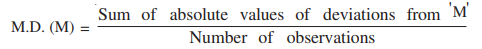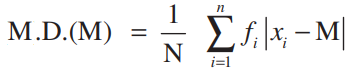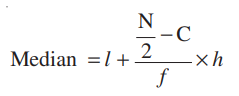In Statistics, Mean deviation is one of the measures of dispersion. This can be obtained from any one of the measures of central tendency. Though, mean deviation from mean and median are the most commonly used statistical considerations. In this article, one can learn what is mean deviation about median, how to find mean deviation about median in case of both grouped and ungrouped data along with examples.

## Mean Deviation About The Median

The mean deviation about a median value ‘M’ is the mean of the absolute values of the deviations of the observations from ‘M’. The mean deviation from ‘M’ is denoted as M.D. (M).

### Mean Deviation About Median Formula

The mean deviation from the median formula is given by:Here, “M” represents the median of the given data. However, we can find the mean deviation from the median for ungrouped and grouped data as explained below.

## How to Calculate Mean deviation from median

The three main steps involved in finding the mean deviation about median either for ungrouped or grouped data are given below:

Step 1: Find the median value for the given data values.

Step 2: Now, subtract the median value from each of the data values given and consider the absolute deviations here.

Step 3: Finally, calculate the mean of deviations obtained in the previous step.

### Mean Deviation About Median For Ungrouped Data

Let x1, x2, x3,…, xn be n observations. For this data, the formula to find the mean deviation from the median is given as:

$$\large M.D.(M)=\frac{1}{n}\sum_{i=1}^{n}|x_{i}-M|$$

Here,

M = Median of the given data

Let’s have a look at the example given below for better understanding.

Example 1:

Find the mean deviation about the median for the data: 6, 7, 10, 12, 13, 4, 8, 12

Solution:

Given data:

6, 7, 10, 12, 13, 4, 8, 12, 16

Finding the median:

Ascending order of the given data is: 4, 6, 7, 8, 10, 12, 12, 13, 16

Number of data values = 9

Median = (n + 1)/2 th observation

= (9 + 1)/2

= 5th observation

Thus, median = 10

The absolute values of the respective deviations from the median, i.e., |xi − M| are:

|4 – 10|, |6 – 10|, |7 – 10|, |8 – 10|, |10 – 10|, |12 – 10|, |12 – 10|, |13 – 10|, |16 – 10|

= 6, 4, 3, 2, 0, 2, 2, 3, 6

As we know, $$M.D.(M)=\frac{1}{n}\sum_{i=1}^{n}|x_{i}-M|$$

= (6 + 4 + 3 + 2 + 0 + 2 + 2 + 3 + 6)/9

= 28/9

= 3.11

Therefore, the mean deviation about the median for the given data is 3.11.

### Mean Deviation About Median For Grouped Data

For grouped data, there exist two scenarios where we can find the median deviation from the median. They are:

(i) Mean Deviation About Median for Discrete frequency distribution

To find the mean deviation about the median, we need to calculate the median of the given discrete frequency distribution. As we already know how to find the median of data in case of discrete distribution, we can continue finding the mean deviation using the formula given below:Here,

M = Median

xi = Data values

fi = Frequency

N = Sum of the frequencies

Go through the example given below to understand the process clearly.

Example 2: Find mean deviation about the median for the following distribution.

 xi 2 5 6 8 10 12 fi 2 9 12 4 8 5

Solution:

The first step in calculating the median for discrete distribution is to arrange the data in ascending order.

As the given data is already in ascending order,we can find the median as:

 xi 2 5 6 8 10 12 fi 2 9 12 4 8 5 cf 2 11 23 27 35 40

N = 40 (even)

N/2 = 40/2 = 20

Median will be the average of 20th and 21st observations.

However, these observations lie in the cumulative frequency (cf) 23, for which the corresponding observation is 6.

Median = (6 + 6)/2 = 6

Let’s prepare another table for getting the absolute deviations.

 |xi – M| 4 1 0 2 4 6 fi 2 9 12 4 8 5 fi |xi – M| 8 9 0 8 32 30

We know that,

$$M.D.(M)=\frac{1}{N}\sum_{i=1}^{n}f_{i}|x_{i}-M|$$

= (8 + 9 + 0 + 8 + 32 + 30)/40

= 87/40

= 2.175

Therefore, the mean deviation about the median for the given distribution is 2.175.

(ii) Mean Deviation About Median for Continuous frequency distribution

The mean deviation from the median of continuous frequency distribution can be calculated using the following formula.Here,

xi = Mid-point of the class interval

fi = Frequency

N = Sum of the frequencies

M = Median

For continuous frequency distribution, the formula of median is given as:Here,

l = Lower limit of median class

N = Number of observations (sum of the frequencies)

C = Cumulative frequency of class preceding the median class

f = Frequency of median class

h = Class size (assuming class size to be equal)

Given below is the solved example to learn how to find the mean deviation about median for continuous distribution of data.

Example 3: Calculate the mean deviation about the median for the following distribution.

 Class interval 10 – 20 20 – 30 30 – 40 40 – 50 50 – 60 60 – 70 70 – 80 Frequency 2 3 8 14 8 3 2

Solution:

Let us calculate the median and mean deviation for the given data.

 Class interval Frequency (fi) Cumulative frequency Mid-points (xi) |xi – M| fi |xi – M| 10 – 20 2 2 15 30 60 20 – 30 3 5 25 20 60 30 – 40 8 13 35 10 80 40 – 50 14 27 45 0 0 50 – 60 8 35 55 10 80 60 – 70 3 38 65 20 60 70 – 80 2 40 75 30 60 Total ∑fi = 40 ∑fi |xi – M| = 400

N = ∑fi = 40

N/2 = 40/2 = 20

The cumulative frequency greater than or equal to N/2 is 27, for which the corresponding class interval is 40 – 50.

Median class = 40 – 50

Thus,

l = 40

N = 40

C = 13

f = 14

h = 10

Median = l + [(N/2 – C)/f] × h

= 40 + [(20 – 13)/14] × 10

= 40 + (7/14) × 10

= 40 + 5

= 45

Therefore, medan (M) = 45

From the above table,

∑fi |xi – M| = 400

N = ∑fi = 40

Hence, the required mean deviation = 400/40 = 10